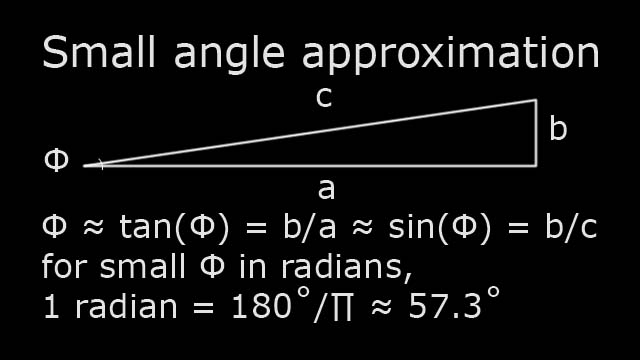# See Moon landing sites from earth?

How powerful a telescope is needed to see the things left behind at the Apollo Moon landing sites from here on earth? There are several parts to the answer. If you want to skip the details, here is the result.

• Magnification 1.2 million X with a 4mm eyepiece
• Focal length 4.8 km (3 miles)
• Objective diameter 2.24 km (1.4 miles)
• Location in low earth orbit

A telescope of this size is about 100 times larger than any we have built. It is easier to send a much smaller telescope closer to the Moon as we have with the Lunar Reconnaissance Orbiter and perhaps even easier to return in person...

### Magnification

Human eyes can only detect objects that are about 1 arc minute (1/60 of a degree in size). To calculate the size of the details we need to see, we will solve the triangle defined by our eye, the distance to the Moon, and the distance we want to resolve. We will use units of Radians for our angles, because this makes the calculation simpler.

Lets assume that we would like to see the Apollo left behinds with details of about 10 cm (about 4 inches or 0.1 meters). The Moon is about 400,000 km away (4x10^8 meters). We have two sides of a right triangle and can solve it for the angle that our eye must see. A handy simplification is the small angle approximation:Using this equation, the angle that we must see is:

We must magnify this tiny angle to be large enough for our eye to see, 1 arc minute ( 1/(60 * 57.3) = 0.0003 R)

magnification = 0.0003 / 2.5x10^(-10) = 1.2x10^6 power

Our telescope must have a magnification of at least 1.2 million times! We can calculate the focal length of our telescope using a magnification equation and our eyepiece focal length. About 4mm is the shortest eyepiece that we can practically use in a telescope.

### Telescope sizeUsing this formula our telescope focal length must be:

fo = fe * magnification = 4x10^(-3) * 1.2x10^6 = 4.8x10^3 = 4.8 km (3 miles)

In addition to magnifying power, we need to consider the diffraction limited resolution of this telescope. The wave nature of light causes it to be diffracted when it passes through the aperture that defines the telescopes objective size. This puts a practical limit on the magnification of a telescope with a given size aperture before the image becomes too blurry. The diameter of the telescope's objective (lens or mirror) is determined by this diffraction limit.

The physics of diffraction are well understood theoretically and empirically. Dawes' limit is one often used measure of diffraction limited resolution:

R=116/D with Resolution in arc sec, and apperture D in mm
1 arc sec = 1/(57.3 * 60 * 60) Radians = 1/206280 R = 4.85x10^(-6) R
R = 5.6x10^(-4)/D with D in mm and R in radians
D = 5.6x10^(-7)/R with D in m and R in radians

Substituting our required resolution of 2.5x10^(-10) radians
D = 5.6x10^(-7)/2.5x10^(-10) = 2240 m = 2.24 km (1.4 miles)

### Location

Turbulence in the air limits the detail that we can see to about 1 arc second. This will certainly spoil our view of the Apollo sites. The telescope needs to be above the air in low earth orbit to give us a clear view.

Content created: 2018-02-10